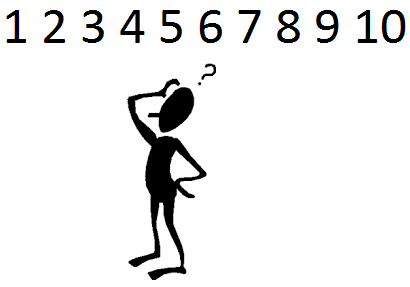﻿ Number Guessing Game Built with PHP- Guess a Number from 1 to 10 ﻿# Guessing Game- Guess a Number From 1 to 10Guess a Number Between 1 and 10:

Result:

Above is a guessing game created in PHP.

With the game above, a user guesses a number from 1 to 10. The computer generates a random number from 1 to 10. If the number that the user enters matches the number that the computer generates, the user has guessed the correct number. If the number that the user has entered does not match the number that the computer has generated, the user is told that this is the incorrect number and to try again.

Each entry that a user enters is independent of all other entries, meaning that the computer generates a new random number each time an attempt is tried. The numbers keep switching. The computer generates a new random number each time the submit button is clicked. So if you enter '4' and the computer generates a '5' and then you enter a '5', the computer doesn't store that same 5. The next time you enter a number, it can and many times will be a totally new number.

So how do we go about building this guessing game?

So the basics of the PHP code is that we use the mt_rand() function, which can generate a new number each time. We specify in this function that we want the random number to range from 1 to 10. So the computers selects any random number from 1 to 10 each time a guess is tried. We then extract what the user has entered in the search box. If the number that the user entered matches the number the computer has generated, the user has guessed the correct function. If not, the user tries again.

### Full Code

The full code to build this PHP game above is shown below.

So the first block of code is PHP code. Here, in this block, we get the number that the user has entered in the search box. We also extract whether the submit button has been clicked or not. We then generate a random number using the mt_rand() function and store in the \$randomnumber variable. The mt_rand function returns a random number (of course it's not truly random being that it's made up of computer algorithms but good enough to work for this). In the mt_rand() function, we specify the range of numbers we want returned. Being that we want a number from 1 to 10 returned in the random function, we specify these 2 parameters in the function.

The next block of code creates the HTML form element. The action attribute is set to "" (null) because we want the information extracted from this form to stay on this page. If we wanted the information extracted from this form to be redirected to another page, we want this specify this page in this attribute. The method is POST.

We then write the line, Guess a Number Between 1 and 10. Right beside this, we put an HTML text box. If the submit button is clicked and the number entered is not between 1 and 10, we output the line, "The number must be between 1 and 10". This is so that if the user enters 0 or a negative number or a number greater than 10, he or she will know that this not an acceptable input. The number must be between 1 and 10.

Next we have the result on the next line. If the submit button is pressed and the number is between 1 and 10 and the number entered is not equal to the number the computer generated, we output that this guess is incorrect and what the correct number was and to try again. Else, we output that the number entered is the correct guess and that the user got it right.

It's very simple code. It's able to create a somewhat fun game. And this is all that is required to create a guessing game using PHP.